# Check If Any Cell in Range is a Number – Excel & Google Sheets

This tutorial will demonstrate how to check if any cell in a range contains a number in Excel and Google Sheets.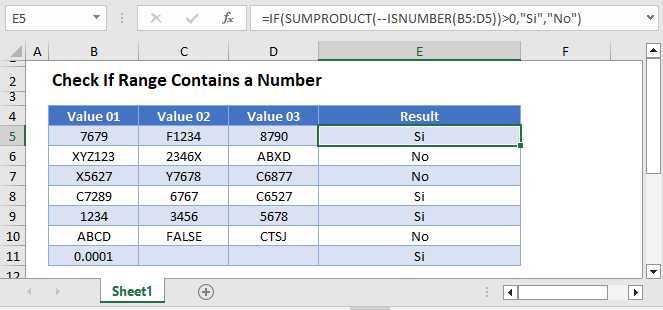## Check if Range Contains a Number

To test if any cell in a range contains a number, we will use the ISNUMBER and SUMPRODUCT Functions.

### ISNUMBER Function

The ISNUMBER Function does exactly what its name implies. It tests if a cell is a number, outputting TRUE or FALSE.

``=ISNUMBER(A2)``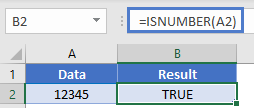### SUMPRODUCT Function

The ISNUMBER Function only tests a single cell, so we must find a way to test the entire range. This is where the SUMPRODUCT Function comes in.  The SUMPRODUCT Function sums together an array of values. So if we can test each cell individually, outputting a 1 (TRUE) or 0 (FALSE) if each cell as text, then we can sum the values together. If the sum is greater than 0 than we know at least one cell contained a number.

First, here is the final calculation:

``=SUMPRODUCT(--ISNUMBER(A2:C2))>0``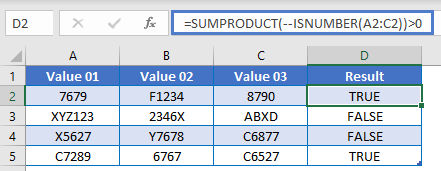Now let’s walk through it.

``````=SUMPRODUCT(--ISNUMBER(A2:C2))>0

=SUMPRODUCT(--{TRUE, FALSE, TRUE})>0

=SUMPRODUCT({1, 0, 1})>0

=2>0

=TRUE``````

Note: the double negatives converts the TRUE/FALSE into 1 / 0. Alternatively you could multiply the ISNUMBER Function by *1

``=SUMPRODUCT(ISNUMBER(A2:C2)*1)>0``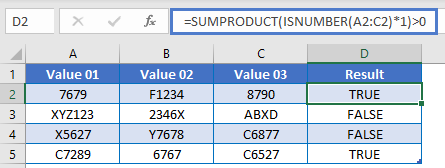Instead of outputting TRUE / FALSE, you can use an IF Statement to output other text or calculations:

``=IF(SUMPRODUCT(--ISNUMBER(A2:C2))>0, "Yes", "No")``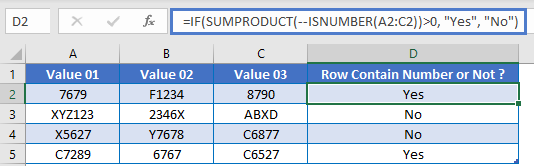## Google Sheets – Check If Any Cell in Range is a Number

All of the above examples work exactly the same in Google Sheets as in Excel.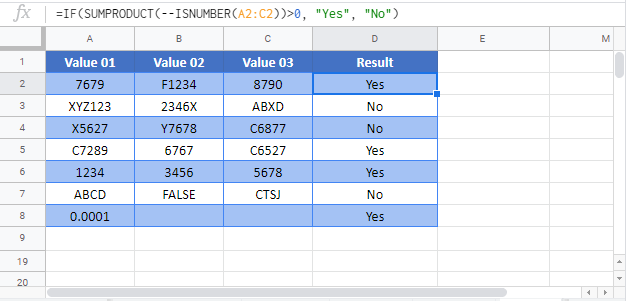### Excel Practice Worksheet

Practice Excel functions and formulas with our 100% free practice worksheets!